## A 6.00 g sample of an optically pure compound was dissolved in 40.0 mL of CCl4. The observed rotation was +3.30 °, measured in a 10.0 cm (1.

Question

A 6.00 g sample of an optically pure compound was dissolved in 40.0 mL of CCl4. The observed rotation was +3.30 °, measured in a 10.0 cm (1.00 dm) polarimeter tube.

in progress 0
6 months 2021-07-17T23:41:42+00:00 1 Answers 2 views 0

1. The question is incomplete, the complete question is:

If a 6.00 g sample of an optically pure compound was dissolved in 40.0 mL of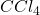and the observed rotation was +3.30°, measured in a 10.0 cm (1.00 dm) polarimeter tube, how would one determine the specific rotation of the pure compound?

Answer: The specific rotation of the pure compound is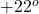Explanation:

To calculate the specific rotation of a pure compound, we use the equation: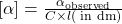where,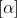= specific rotation of a pure compound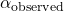= observed rotation of the compound = [ex]+3.30^o[/tex]

C = concentration in g/mL = 6.00 g/40 mL = 0.15 g/mL

l = path length = 1.00 dm

Putting values in above equation, we get: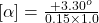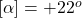Hence, the specific rotation of the pure compound is